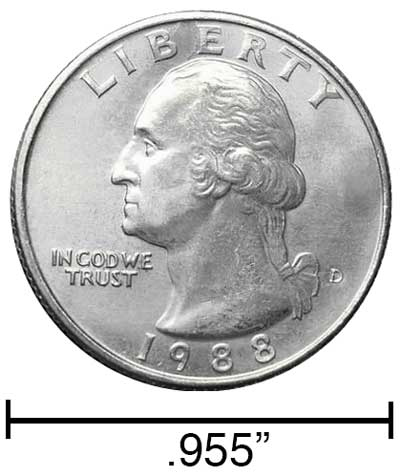# Inches to Miles Conversion

Enter the length in inches below to get the value converted to miles.

/
Results in Miles:1 in = 1.5783E-5 mi
Do you want to convert miles to inches?

## How to Convert Inches to MilesTo convert an inch measurement to a mile measurement, divide the length by the conversion ratio.

Since one mile is equal to 63,360 inches, you can use this simple formula to convert:

miles = inches ÷ 63,360

The length in miles is equal to the inches divided by 63,360.

For example, here's how to convert 50,000 inches to miles using the formula above.
50,000" = (50,000 ÷ 63,360) = 0.789141 mi

Our inch fraction calculator can add inches and miles together, and it also automatically converts the results to US customary, imperial, and SI metric values.

## Inches

An inch is a unit of linear length measure equal to 1/12 of a foot or 1/36 of a yard. Because the international yard is legally defined to be equal to exactly 0.9144 meters, one inch is equal to 2.54 centimeters.

The inch is a US customary and imperial unit of length. Inches can be abbreviated as in; for example, 1 inch can be written as 1 in.

Inches can also be denoted using the symbol, otherwise known as a double-prime. Often a double-quote (") is used instead of a double-prime for convenience. A double-prime is commonly used to express 1 in as 1″.The standard ruler has 12", and is a common measuring tool for measuring inches. They are also often measured using tape measures, which commonly come in lengths from 6' - 35'. Other types of measuring devices include scales, calipers, measuring wheels, micrometers, yardsticks, and even lasers.

## Miles

The mile is a linear measurement of length equal to exactly 1,609.344 meters. One mile is also equal to 5,280 feet or 1,760 yards.

The mile is a US customary and imperial unit of length. Miles can be abbreviated as mi, and are also sometimes abbreviated as m. For example, 1 mile can be written as 1 mi or 1 m.

We recommend using a ruler or tape measure for measuring length, which can be found at a local retailer or home center. Rulers are available in imperial, metric, or combination with both values, so make sure you get the correct type for your needs.

Need a ruler? Try our free downloadable and printable rulers, which include both imperial and metric measurements.

## Inch to Mile Conversion Table

Inch measurements converted to miles
Inches Miles
1" 0.000015783 mi
2" 0.000031566 mi
3" 0.000047348 mi
4" 0.000063131 mi
5" 0.000078914 mi
6" 0.000094697 mi
7" 0.00011 mi
8" 0.000126 mi
9" 0.000142 mi
10" 0.000158 mi
100" 0.001578 mi
1,000" 0.015783 mi
10,000" 0.157828 mi
100,000" 1.5783 mi

## References

1. National Institute of Standards and Technology, Checking the Net Contents of Packaged Goods, Handbook 133 - 2019 Edition, https://nvlpubs.nist.gov/nistpubs/hb/2019/NIST.HB.133-2019.pdf